Parallel categories plot of selected configurations. Numerical parameters are discretized to maximum n_bins intervals. To visualize configurations of other iterations these must be provided setting the argument iterations, groups of configurations of different iterations are shown in different colors. Specific configurations can be selected providing their ids in the id_configurations argument.

parallel_cat(
irace_results,
id_configurations = NULL,
param_names = NULL,
iterations = NULL,
by_n_param = NULL,
n_bins = 3,
filename = NULL
)

## Arguments

irace_results

The data generated when loading the .Rdata file created by irace (or the filename of that file).

id_configurations

Configuration ids to be included in the plot. Example: c(20,50,100,300,500,600,700)

param_names

(character()) Parameters to be included in the plot. Example: c("algorithm","alpha","rho","q0","rasrank").

iterations

Numeric vector, iterations from which configuration should be obtained (example: iterations = c(1,4,5))

by_n_param

Numeric (optional), maximum number of parameters to be displayed.

n_bins

Numeric (default 3), number of intervals to generate for numerical parameters.

filename

(character(1)) File name to save the plot, for example "~/path/example/filename.png".

## Value

parallel categories plot

## Details

The parameters to be included in the plot can be selected with the param_names argument. Additionally, the maximum number of parameters to be displayed in one plot. A list of plots is returned by this function in several plots are required to display the selected data.

parallel_coord() parallel_coord2()

## Examples

iraceResults <- read_logfile(system.file(package="irace", "exdata",
"irace-acotsp.Rdata", mustWork = TRUE))
parallel_cat(iraceResults)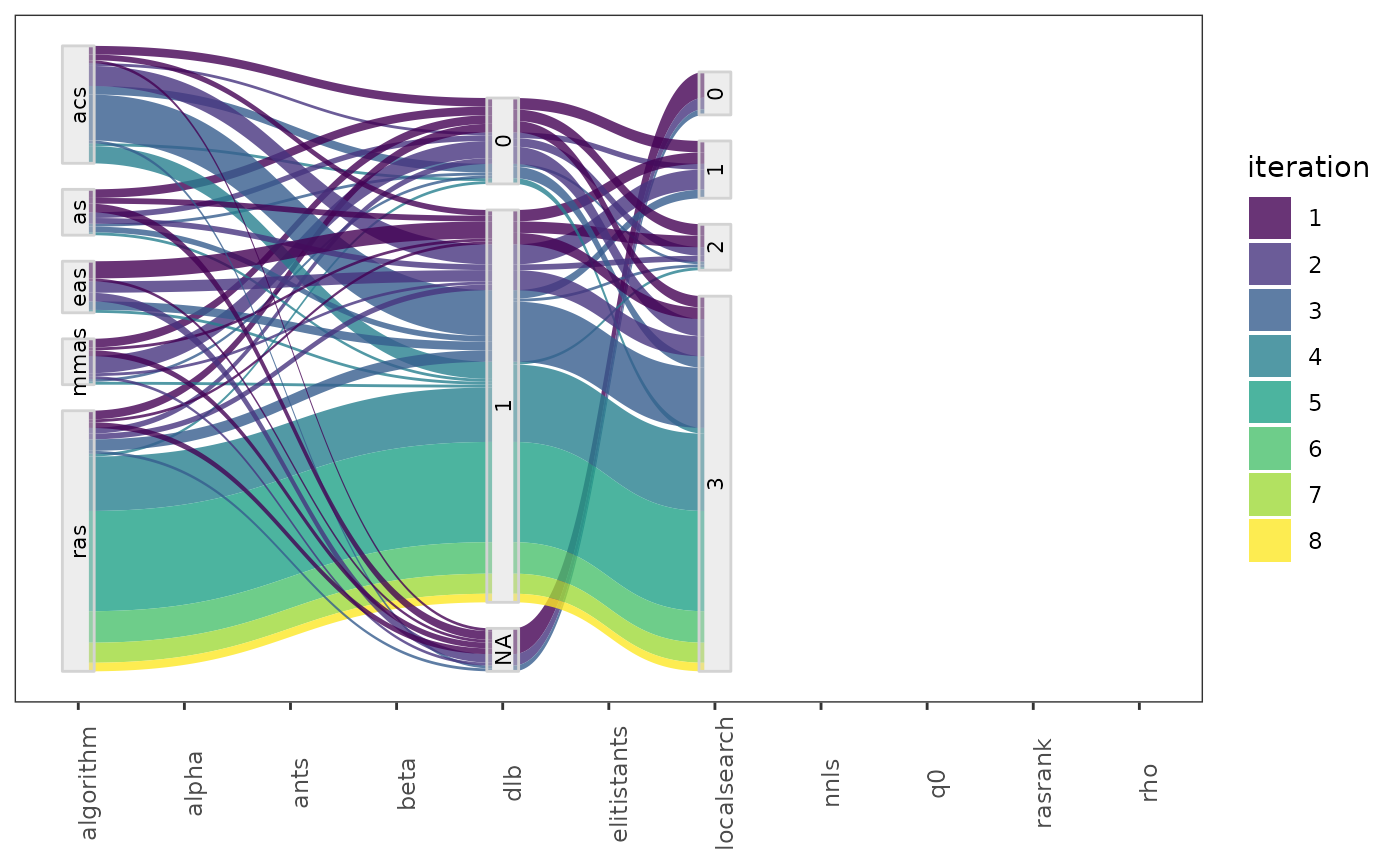# \donttest{
parallel_cat(iraceResults, by_n_param = 6)
#> []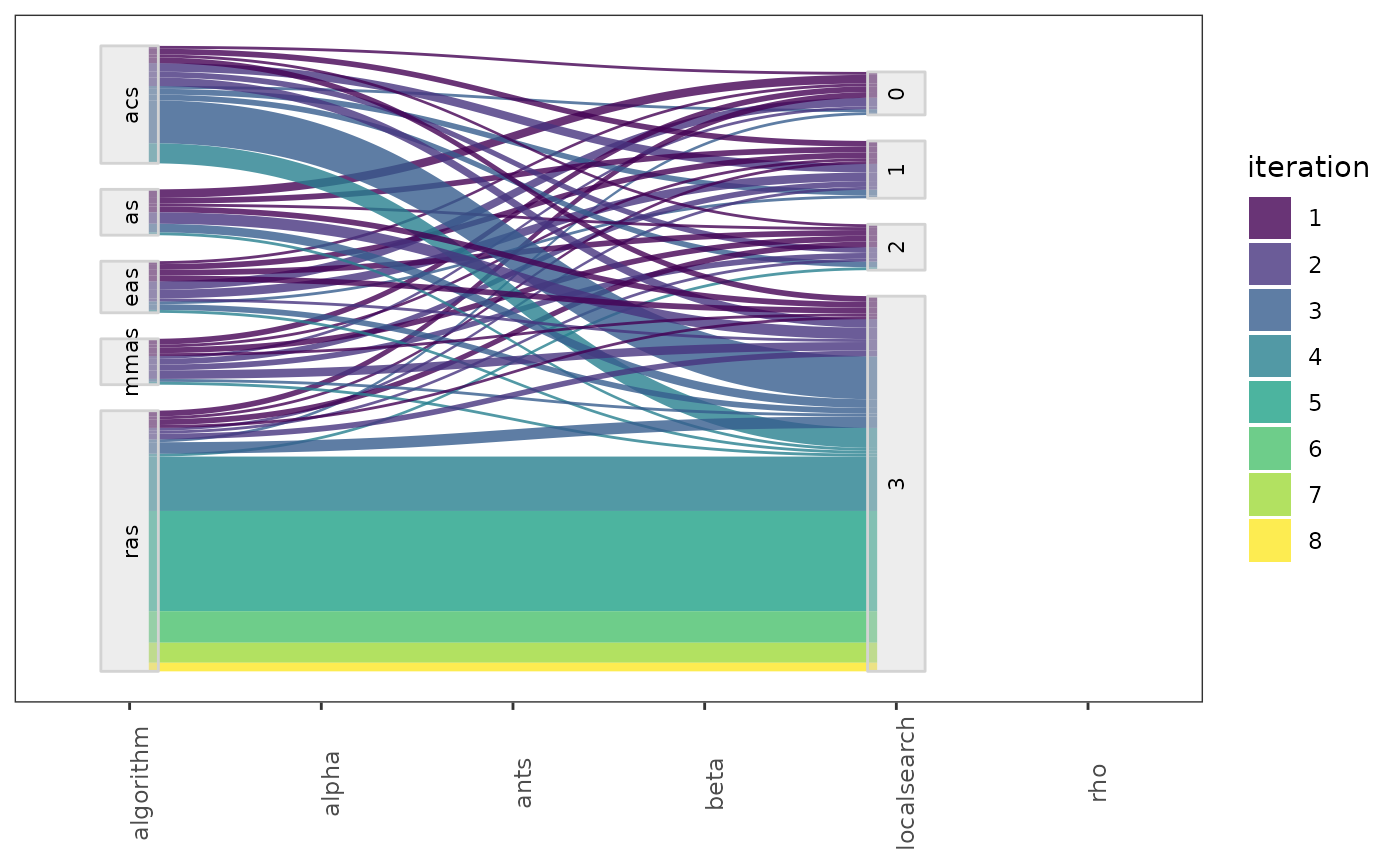#>
#> []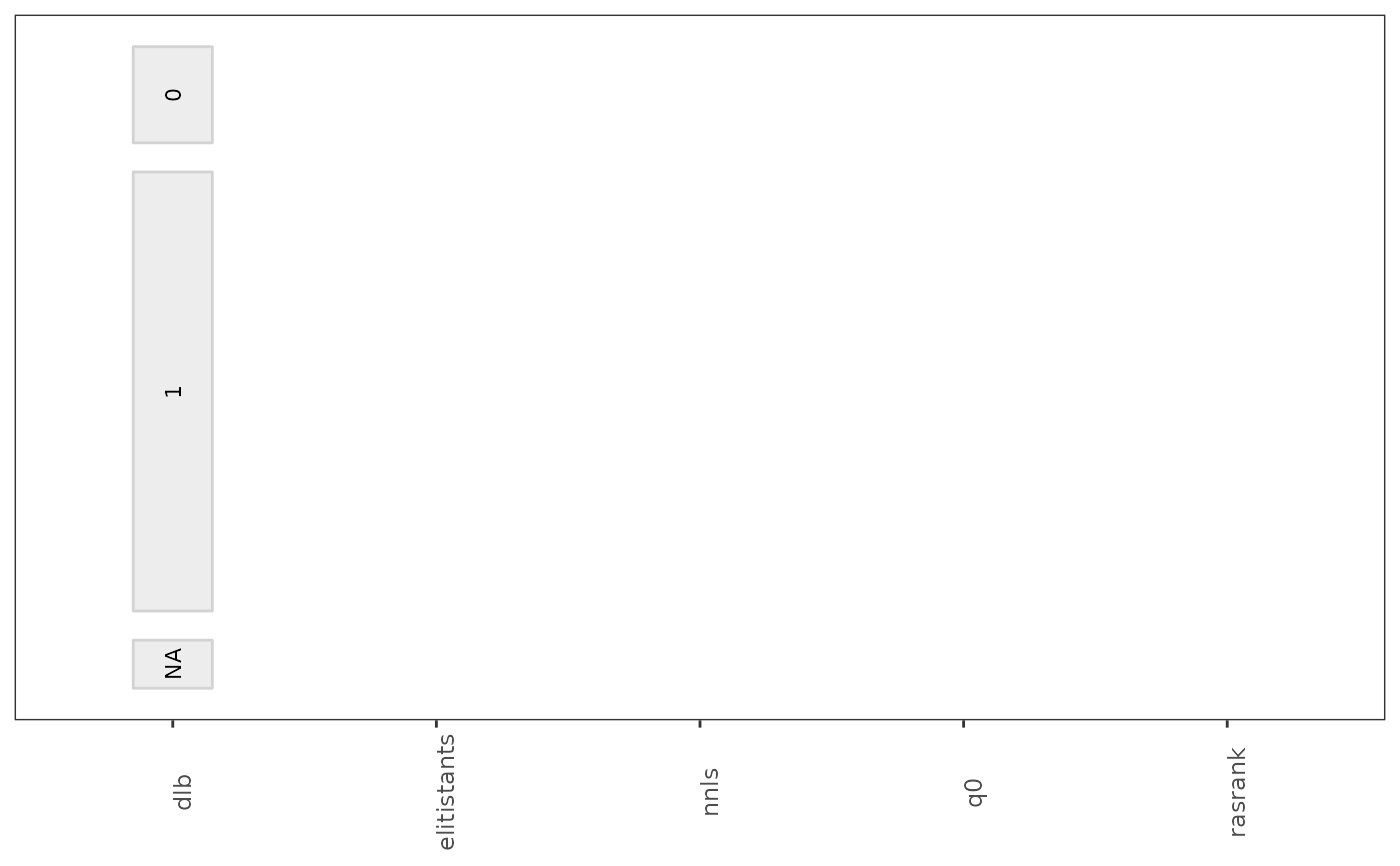#>
parallel_cat(iraceResults, id_configurations = c(20, 50, 100))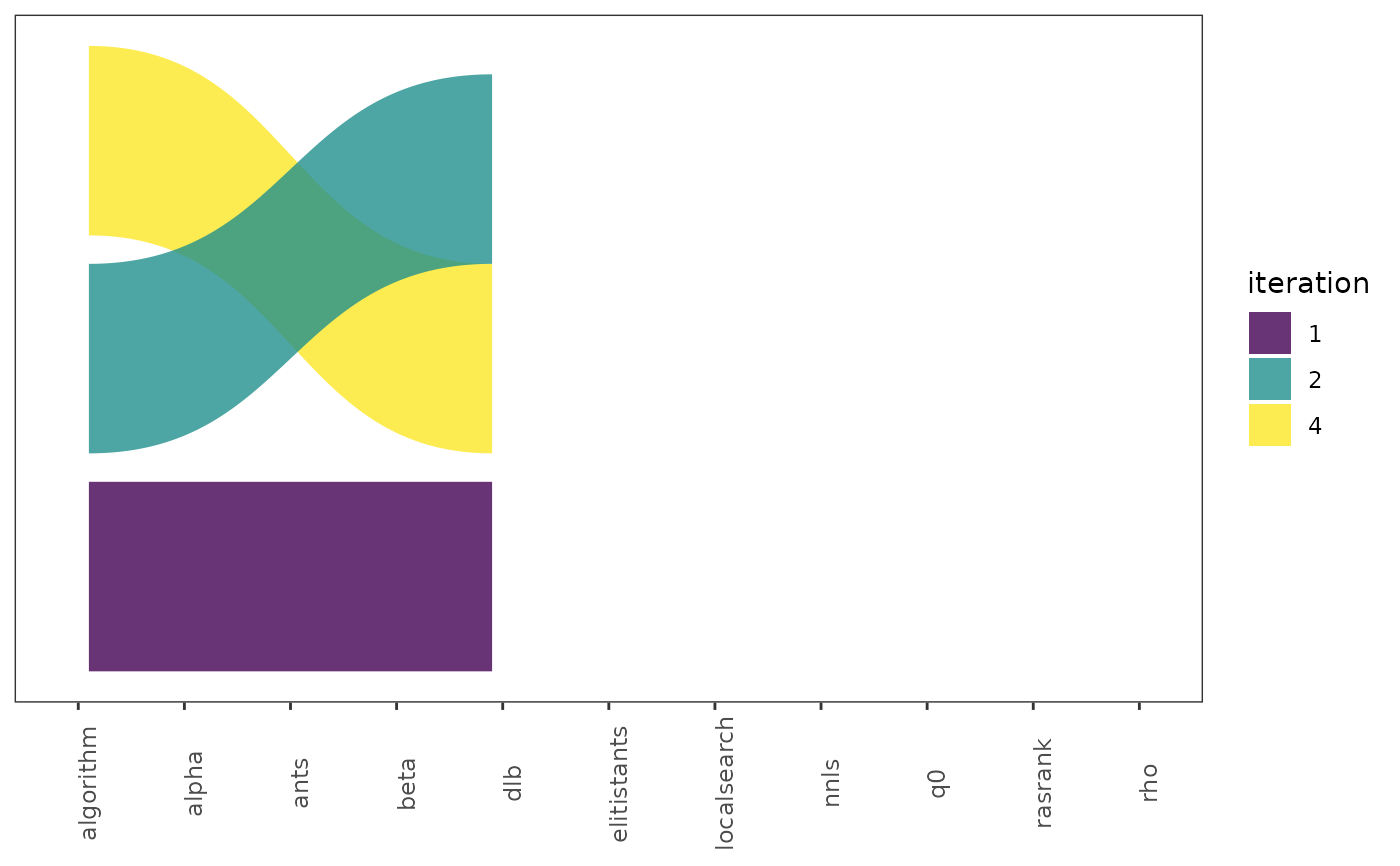parallel_cat(iraceResults, param_names = c("algorithm", "alpha", "rho", "q0", "rasrank"))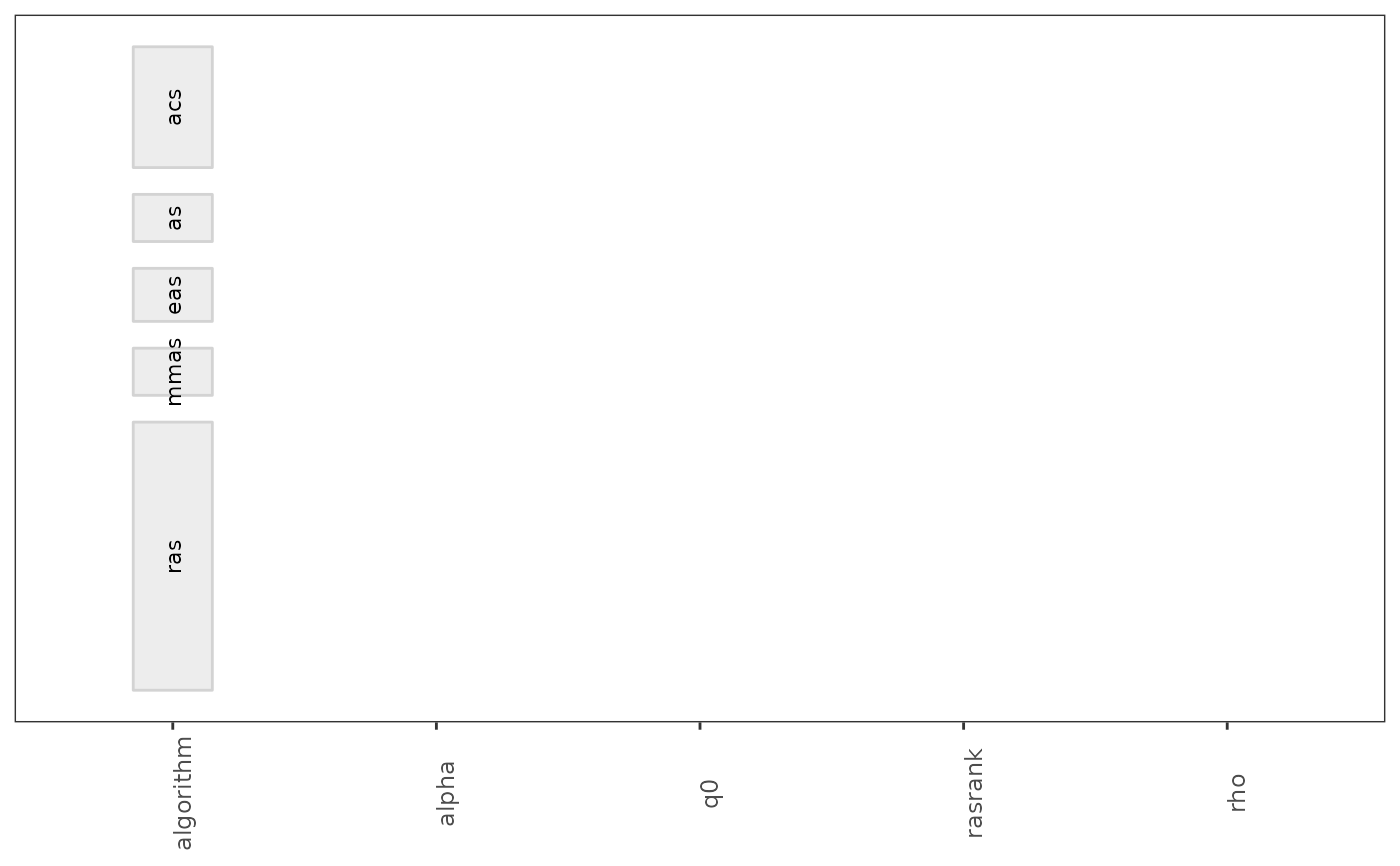parallel_cat(iraceResults, iterations = c(1, 4, 6), n_bins=4)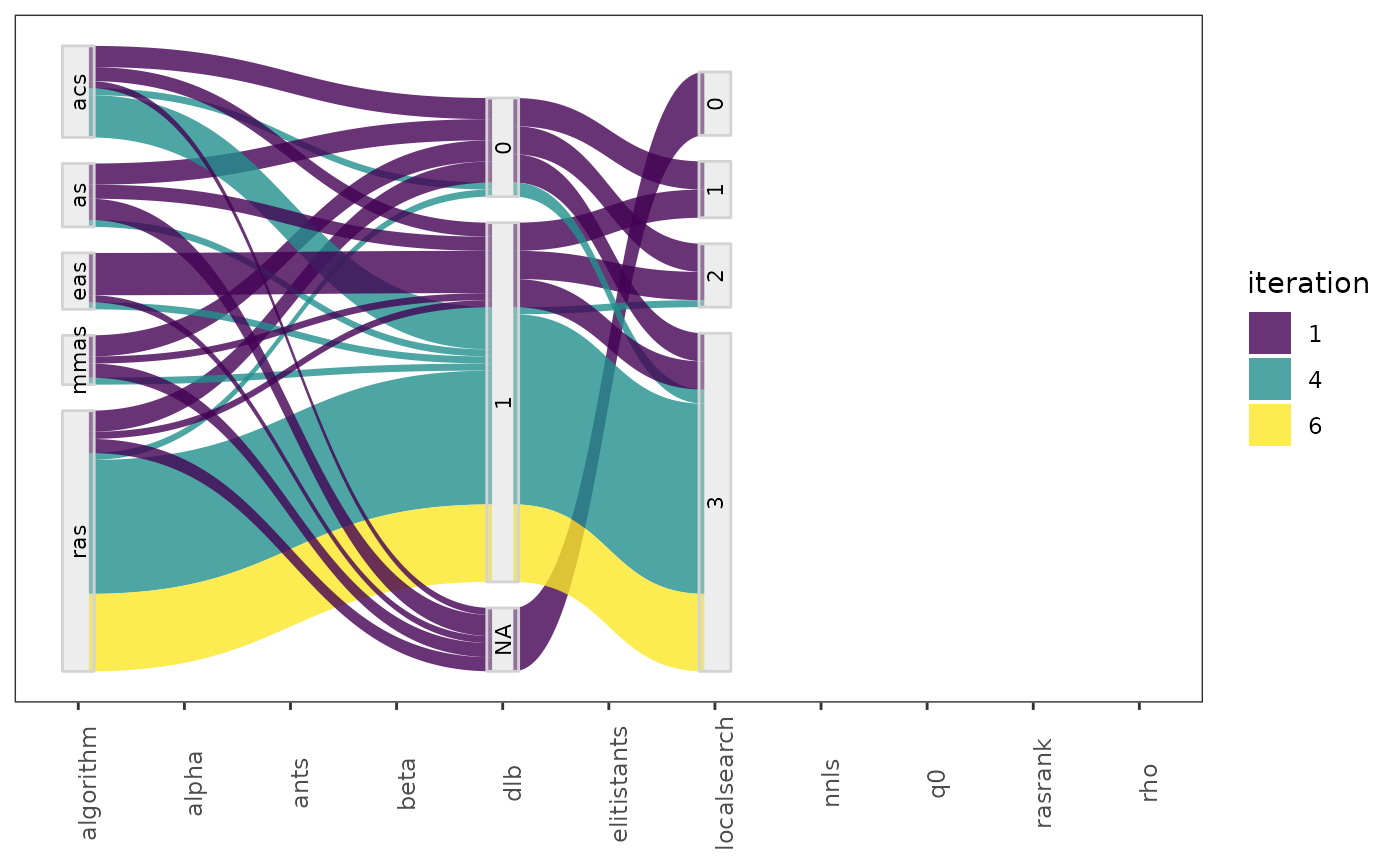# }